# 使用开源概率编程语言 Pyro 对截尾时间 - 事件数据进行建模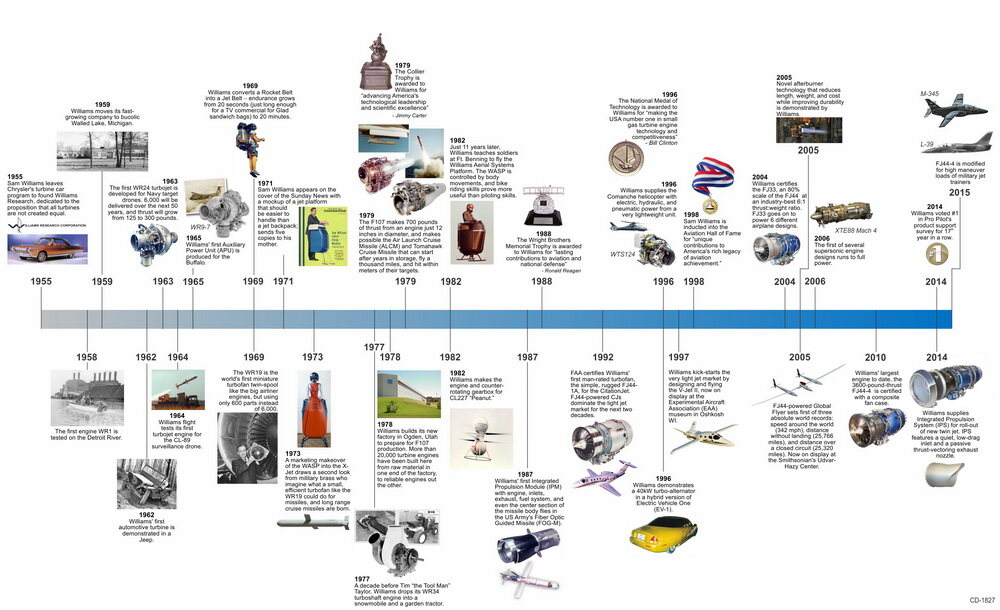$(T_i,L_i)$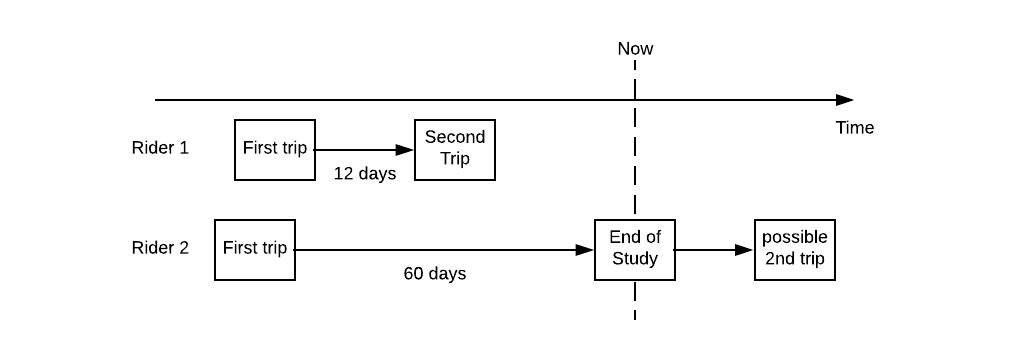## 用于统计建模的 Pyro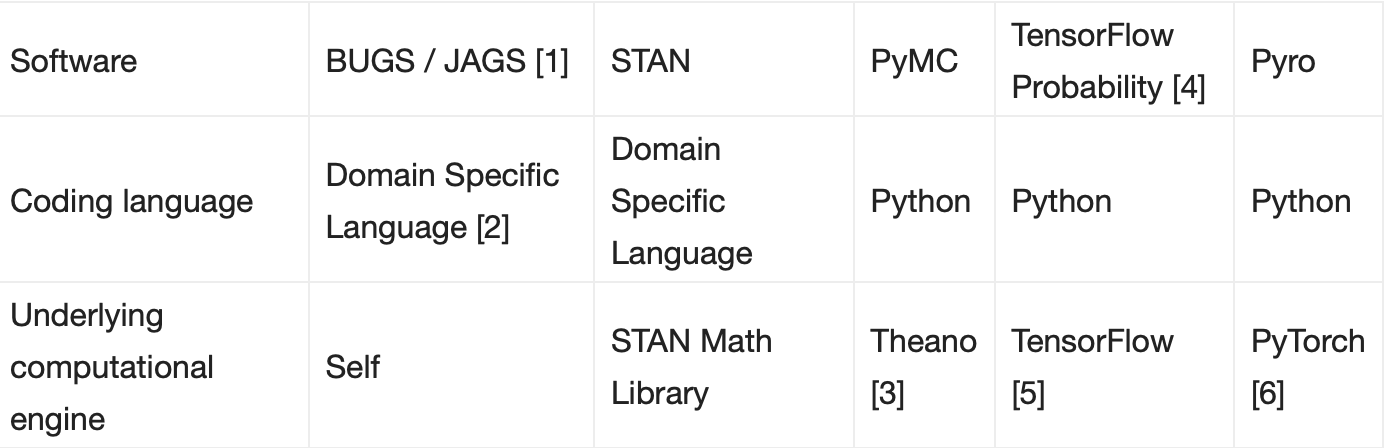1. BUGS/JAGS 是概率编程早期的例子。在统计领域，它们已经被积极开发和使用了 20 多年。
2. 但是，BUGS/JAGS 主要是从头设计和开发的。因此，模型规范是用它们特定于域的语言完成的。此外，概率程序开发人员需要从 R 和 MATLAB 中的包装器中调用 BUGS/JAGS。用户必须在编码语言和文件之间来回切换，不太方便。
3. PyMC 依赖于 Theano 后端。但是，Theano 项目最近停止了。
4. TensorFlow Probability（TFP）最初作为一个名为 Edward 的项目启动。该 Edward 项目已纳入 TFP 项目。
5. TFP 使用 TensorFlow 作为其计算引擎。因此，其仅支持静态计算图。
6. Pyro 使用 PyTorch 作为计算引擎，因此支持动态计算图。这使得用户能够在数据流方面指定不同的模型，非常灵活。

## 对截尾的时间 - 事件数据建模

### 模型定义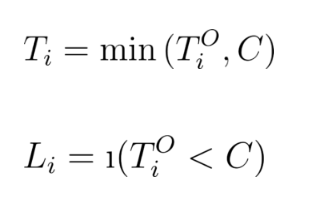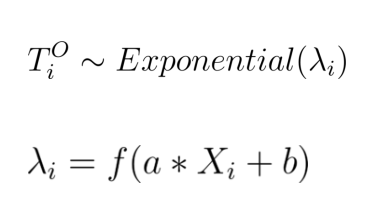### 生成人工数据

import pyro import torch import seaborn as sns import pyro.distributions as dist from pyro import infer, optimfrom pyro.infer.mcmc import HMC, MCMCfrom pyro.infer import EmpiricalMarginal assert pyro.__version__.startswith('0.3')

n = 500a = 2b = 4c = 8 x = dist.Normal(0, 0.34).sample((n,)) # Note  link = torch.nn.functional.softplus(torch.tensor(a*x + b))# note below, param is rate, not meany = dist.Exponential(rate=1 / link).sample() truncation_label = (y > c).float() y_obs = y.clamp(max=c) sns.regplot(x.numpy(), y.numpy())sns.regplot(x.numpy(), y_obs.numpy()) ## Note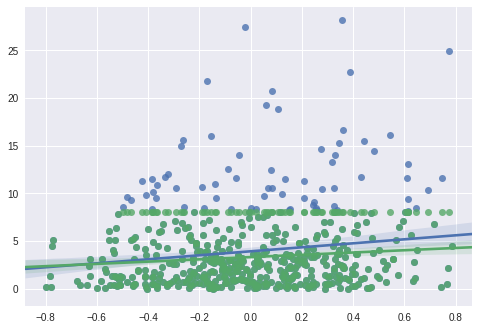### 构建模型

def model(x, y, truncation_label): ## Note    a_model = pyro.sample("a_model", dist.Normal(0, 10)) ## Note    b_model = pyro.sample("b_model", dist.Normal(0, 10))    link = torch.nn.functional.softplus(a_model * x + b_model) ## Note      for i in range(len(x)):    y_hidden_dist = dist.Exponential(1 / link[i]) ## Note          if truncation_label[i] == 0:       ## Note        y_real = pyro.sample("obs_{}".format(i),                            y_hidden_dist,                           obs = y[i])    else:      ## Note       truncation_prob = 1 - y_hidden_dist.cdf(y[i])      pyro.sample("truncation_label_{}".format(i),                   dist.Bernoulli(truncation_prob),                   obs = truncation_label[i])

• Note 1：总的来说，模型函数描述的是数据生成的过程。这个示例模型函数告诉我们如何从输入的矢量 x 生成 y 或 truncation_label。
• Note 2：我们指定这里﻿$a$ 和﻿$b$ 的先验分布，并利用 pyro.sample 函数对它们采样。Pyro 在 PyTorch 项目和 Pyro 项目中都有大量的随机分布。
• Note 3: 在这里，我们把输入﻿$x$，﻿$a$ 和﻿$b$ 接入用变量 link 表示的﻿$\lambda$ 矢量。
• Note 4：我们利用带有尺度参数矢量链接的指数分布来指定真实时间 - 事件﻿$T^O_i$ 的分布。
• Note 5：对于观察 i，如果我们观察到时间 - 事件数据，那么我们把它和实际观察 y[i] 进行对比。
• Note 6：如果对于观察﻿$i$，数据是截尾的，那么截断标签（这里等于 1）遵循伯努利分布。在﻿$T_i$ 点，观察到截断数据的概率是﻿$T^O_i$ 的 CDF。我们从伯努利分布中采样，并将其与 truncation_label[i] 的实际观察结果进行对比。

### 用哈密顿•蒙特•卡罗方法（Hamiltonian Monte Carlo，简称 HMC）计算推理

pyro.clear_param_store() # note  hmc_kernel = HMC(model,                 step_size = 0.1,                  num_steps = 4)  # Note  mcmc_run = MCMC(hmc_kernel,                 num_samples=5,                warmup_steps=1).run(x, y, truncation_label)  # Note  marginal_a = EmpiricalMarginal(mcmc_run,                                sites="a_model")  # Note  posterior_a = [marginal_a.sample() for i in range(50)]  sns.distplot(posterior_a)

def model(x, y, truncation_label):  a_model = pyro.sample("a_model", dist.Normal(0, 10))   b_model = pyro.sample("b_model", dist.Normal(0, 10))    link = torch.nn.functional.softplus(a_model * x + b_model)     with pyro.plate("data"):    y_hidden_dist = dist.Exponential(1 / link)         with pyro.poutine.mask(mask = (truncation_label == 0)):       pyro.sample("obs", y_hidden_dist,                  obs = y)          with pyro.poutine.mask(mask = (truncation_label == 1)):      truncation_prob = 1 - y_hidden_dist.cdf(y)      pyro.sample("truncation_label",                   dist.Bernoulli(truncation_prob),                   obs = torch.tensor(1.))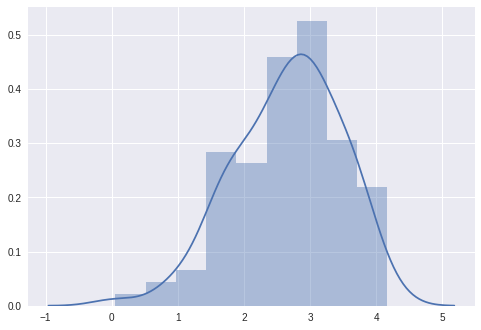### 利用变分推理加速估计

guide = AutoMultivariateNormal(model) 

### 训练模型并推断结果

pyro.clear_param_store()  adam_params = {"lr": 0.01, "betas": (0.90, 0.999)}optimizer = optim.Adam(adam_params) svi = infer.SVI(model,                 guide,                 optimizer,                 loss=infer.Trace_ELBO()) losses = []for i in range(5000):  loss = svi.step(x, y_obs, truncation_label)  losses.append(loss)   if i % 1000 == 0:    print(', '.join(['{} = {}'.format(*kv)                     for kv in guide.median().items()])) print('final result:')for kv in sorted(guide.median().items()):  print('median {} = {}'.format(*kv))

a_model = 0.009999999776482582, b_model = 0.009999999776482582a_model = 0.8184720873832703, b_model = 2.8127853870391846a_model = 1.3366154432296753, b_model = 3.5597035884857178a_model = 1.7028049230575562, b_model = 3.860581874847412a_model = 1.9031578302383423, b_model = 3.9552347660064697final result:median a_model = 1.9155923128128052median b_model = 3.9299516677856445

sns.plt.plot(losses)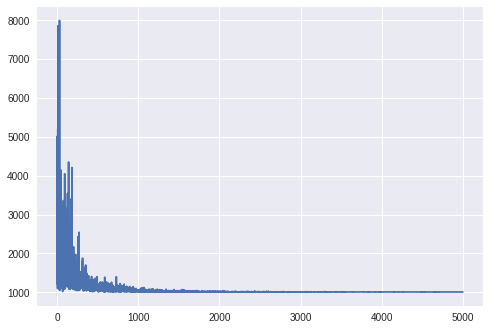N = 1000for name, quantiles in guide.quantiles(torch.arange(0., N) / N).items():  quantiles = np.array(quantiles)  pdf = 1 / (quantiles[1:] - quantiles[:-1]) / N  x = (quantiles[1:] + quantiles[:-1]) / 2  sns.plt.plot(x, pdf, label=name)  sns.plt.legend()sns.plt.ylabel('density')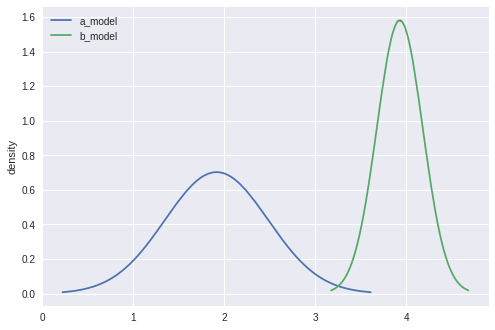## 其他

https://eng.uber.com/modeling-censored-time-to-event-data-using-pyro/